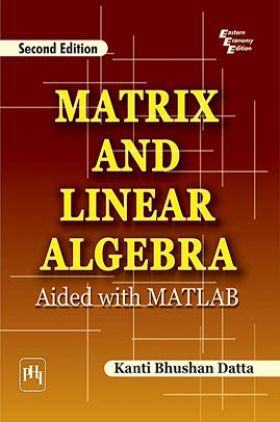# Matrix And Linear Algebra : Aided With Matlab

( 56 customers rating )
12308 Views
MRP : ₹395.00
Price : ₹281.44
You will save : ₹113.56 after 29% Discount
Inclusive of all taxes

Save extra with 2 Offers

Get ₹ 50

Instant Cashback on the purchase of ₹ 400 or above

Product Specifications

 Publisher PHI Learning All Engineering Mathematics books by PHI Learning ISBN 9788120336186 Author: DATTA, KANTI BHUSHAN Number of Pages 636 Edition Second Edition Available Available in all digital devicesAbout The Book Matrix And Linear Algebra

Book Summary:

The present book, renamed Matrix and Linear Algebra: Aided with MATLAB, is a completely re-organized, thoroughly revised and fully updated version of the authors earlier book Matrix and Linear Algebra.

This second edition of the well-received textbook, propelled by the motivation of introducing MATLAB for the study of the numerical aspect of matrix theory, has been developed after taking into account the recent changes in university syllabi, additional pedagogic features needed, as well as the latest developments in the subject areas of Matrix Algebra and Linear Algebra. The use of MATLAB macros throughout the book is the most interesting feature of this edition. Besides, the second edition significantly improves the coverage of all major topics in the two allied subject areas, such as the topics on matrices, determinants, vector spaces, bilinear transformations, and numerical techniques, that were presented in the first edition.

New to the Second Edition

Sections on

MATLAB operations (at the end of most chapters)

Square root, sine, cosine, and logarithm of a matrix

Solution of vector-matrix differential equations

Extensively revised presentation of a section on decomposition of root subspaces

Enhanced discussion of many existing topics

Increased numbers of chapter-end problems and worked-out examples

Many redrawn figures for greater clarity

An exhaustive Solutions Manual for instructors teaching this subject.

The book is highly suitable for undergraduate and postgraduate students of Mathematics, Statistics, and all engineering disciplines. It will also be a useful reference for researchers and professionals in these fields.

Preface
Preface to the First Edition
1 Matrix Algebra
2 Determinants
3 Inverse of a Matrix
4 Rank and Equivalence
5 Vector Space
6 Linear Transformation and Matrices
7 Eigenvalues, Eigenvectors and the Characteristic Equation
8 Bilinear, Quadratic and Hermitian Forms
9 Vector Norms and Matrix Norms
10 Normal Forms
11 Linear Transformations and Normal Forms
12 Function of a Matrix
13 Numerical Linear Algebra
Index

## Engineering Mathematics Linear Algebra PHI Learning books :

Books & Study Materials